Thursday, August 6, 2020
Home > download jstse papers > JSTSE PREVIOUS YEAR QUESTION PAPER 2013-14

# JSTSE PREVIOUS YEAR QUESTION PAPER 2013-14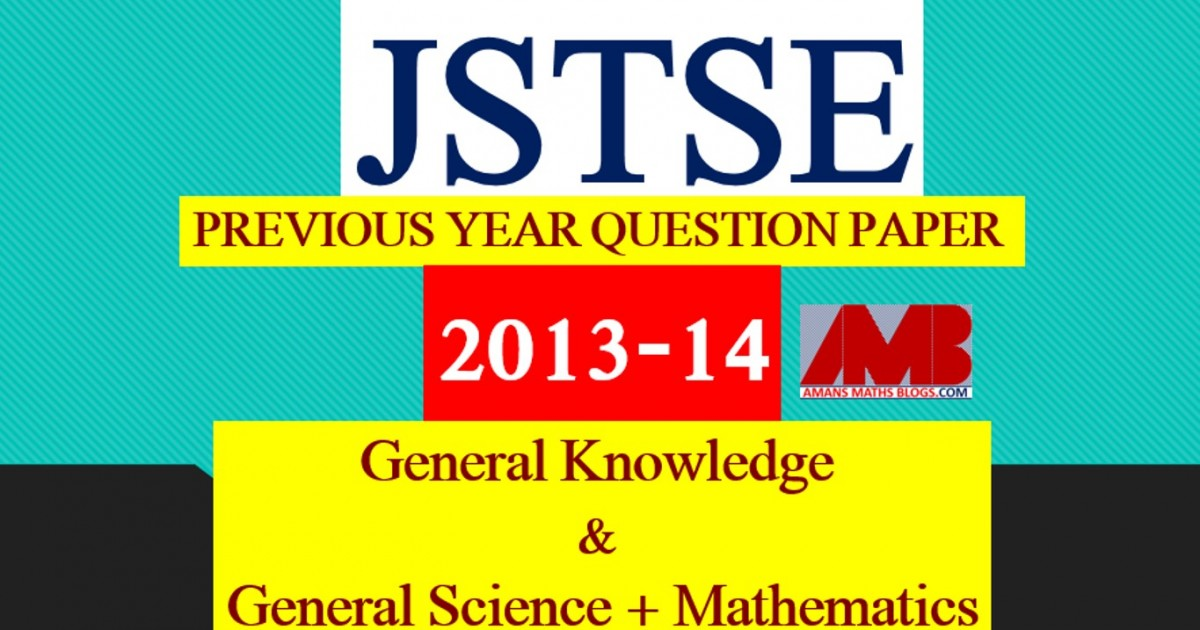# JSTSE PREVIOUS YEAR QUESTION PAPER 2013-14

Junior Science Talent Search Examination (JSTSE) is conducted by Science Branch of DTE of Education, Delhi every year usually in the month of January for the students of recognized schools of Delhi. In this post, you will get JSTSE Previous Year Question Paper 2013-14.

JSTSE
Previous Year Question Papers
2007-082008-092009-102010-112011-12
2012-132013-142014-152015-162016-17
2018-19PhysicsChemistryMathsBiology

In JSTSE, there are two papers: Paper 1 : General Knowledge and Paper 2 :  General Science & Mathematics.

The eligibility of JSTSE exam is any students of recognizing school studying in Class 9th in Govt/Public/KV/Navodaya etc and must have secured 65% marks in Class 8th.

No. of Scholarships:- There are 150 scholarships for JSTSE per year.

Read More : 129 Maths Short Tricks

## General Science + Mathematics

JSTSE Previous Year Question Papers 2013-14 Ques No 1:

If (a + b + c) = 0, then the value of (b + c)2/bc + (c + a)2/ca + (a + b)2/ab is

Options:

A. 0

B. 1

C. 2

D. 3

JSTSE Previous Year Question Papers 2013-14 Ques No 2:

If x2 – 5x + 1 = 0, then the value of x2 + 1/x2 is

Options:

A. 20

B. 27

C. 25

D. -25

JSTSE Previous Year Question Papers 2013-14 Ques No 3:

Factors of (3m2 – 2m)(6 – 3m2 + 2m) – 5 are

Options:

A. (3m + 1)(3m – 5)(m – 1)(m + 1)

B. –(3m + 1)(3m – 5)(m – 1)(m + 1)

C. (3m – 1)(3m + 5)(m – 1)(m + 2)

D. –(3m – 1)(3m – 5)(m – 2)(m + 1)

JSTSE Previous Year Question Papers 2013-14 Ques No 4:

If (x6y-2/x-2y3)-1/2 + (x-1y2/x2y-2)-1/3 = xayb, then the value of (a + b + 1) is

Options:

A. 0

B. 2

C. -1

D. –2

JSTSE Previous Year Question Paper 2013-14 Ques No 5:

In the figure, O is the centre of the circle and OA = CD, then the value of ∠CPD is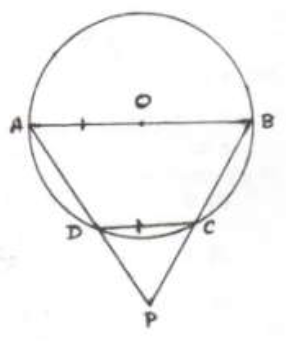Options:

A. 45 degree

B. 30 degree

C. 70 degree

D. 60 degree

JSTSE Previous Year Question Papers 2013-14 Ques No 6:

If a polygon has 27 diagonals, then the number of the sides of the polygon is

Options:

A. 9

B. 10

C. 11

D. 12

JSTSE Previous Year Question Paper 2013-14 Ques No 7:

If x2 + 7ax + 40 and x2 + 2ax – 60 have a common factor, then the value of ‘a’ is

Options:

A. ±1

B. ±2

C. ±3

D. ±4

JSTSE Previous Year Question Papers 2013-14 Ques No 8:

If 642x-1 = 4 x 8x-5, then the value of x is

Options:

A. 17/9

B. 17/10

C. 20/9

D. 9/17

JSTSE Previous Year Question Paper 2013-14 Ques No 9:

If x = 22/3 + 21/3, then

Options:

A. x3 – 6x – 6 = 0

B. x3 + 6x – 6 = 0

C. x3 – 6x + 6 = 0

D. x3 + 6x + 6 = 0

JSTSE Previous Year Question Paper 2013-14 Ques No 10:

In the figure, the area of the shaded region is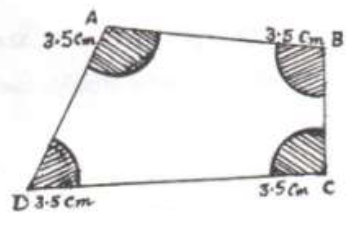Options:

A. 77 cm2

B. 154 cm2

C. 38.5 cm2

D. 90 cm2

JSTSE Previous Year Question Papers 2013-14 Ques No 11:

In the figure, AB = 7.8 cm BC = 5.2 cm and CA = 6.1 cm, then angles x, y and z in ascending orders are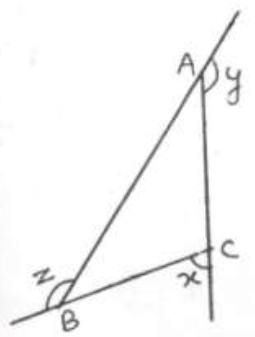Options:

A. x < y < z

B. x > y > z

C. y < z < x

D. x < z < y

JSTSE Previous Year Question Paper 2013-14 Ques No 12:

if a = b2x, b = c3y and c = a3z, then find the value of xyz

Options:

A. 27

B. 1/27

C. 9

D. 1/9

JSTSE Previous Year Question Papers 2013-14 Ques No 13:

If 12, 15, 17, 18, x+2, x+4, 25, 30, 31, 32 are in ascending order and median of the observations is 22, then the value of x is

Options:

A. 20

B. 19

C. 22

D. 23

JSTSE Previous Year Question Paper 2013-14 Ques No 14:

The value of 1/(1 – x) + 1/(1 + x) + 2/(1 + x2) + 4/(1 + x4) + 8/(1 + x8) is

Options:

A. 16/(1 + x16)

B. 8/(1 – x16)

C. 16/(1 – x16)

D. 32/(1 + x18)

JSTSE Previous Year Question Papers 2013-14 Ques No 15:

In the figure ABC, is an equilateral triangle of side 8 cm. Area of the shaded region is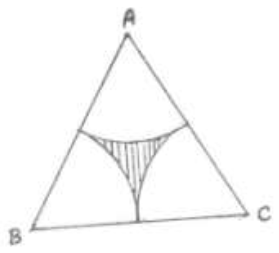Options:

A. 32 – 8π/3 cm2

B. 32 – 16π/3 cm2

C. 16√3 – 8π cm2

D. 32√3 – 16π cm2

JSTSE Previous Year Question Paper 2013-14 Ques No 16:

If x + y = 8, xy = 15, then the value of x4 + x2y2 + y4 is

Options:

A. 34

B. 1156

C. 931

D. 1381

JSTSE Previous Year Question Papers 2013-14 Ques No 17:

The coefficient of x7 in the expansion of (x2 – x + 1)2 + (x2 + x + 1)2 is

Options:

A. 6

B. 5

C. 4

D. 3

JSTSE Previous Year Question Paper 2013-14 Ques No 18:

Mean of 9 observation was found to be 35. Later it on, it was detected that an observation 80 was misread as 8. The correct mean is

Options:

A. 43

B. 42

C. 44

D. 45

JSTSE Previous Year Question Papers 2013-14 Ques No 19:

If (3√2 + 2√3)/(4√2 + 3√3) = a + b√6, then the values of a and b are

Options:

A. a = -6/5 and b = 1/5

B. a = 1/5 and b = 6/5

C. a = 6/5 and b = 1/5

D. a = 6/5 and b = -1/5

JSTSE Previous Year Question Paper 2013-14 Ques No 20:

The value ofOptions:

A. 1

B. 5

C. √5

D. √8

JSTSE Previous Year Question Papers 2013-14 Ques No 21:

A train of length 240 m crosses a platform in 20 seconds. If the speed of the train is 72km/hr, then the length of the platform is

Options:

A. 260 m

B. 160 m

C. 180 m

D. 240 m

JSTSE Previous Year Question Paper 2013-14 Ques No 22:

If (25p + 14q)/(5p + 7q) = 8/3, then p : q is

Options:

A. 3 : 5

B. 2 : 5

C. 2 : 3

D. 5 : 4

JSTSE Previous Year Question Papers 2013-14 Ques No 23:

A farmer can plough a farm in 10 days by working 5 hours a day. In how many days 5 farmers plough 10 such farms working at 5 hours a day?

Options:

A. 20 days

B. 25 days

C. 15 days

D. 24 days

JSTSE Previous Year Question Paper 2013-14 Ques No 24:

The difference between the sides at right angle in a right angled triangle is 14 cm. The area of the triangle is 120 cm2. The perimeter of the triangle is

Options:

A. 50

B. 36

C. 60

D. 34

JSTSE Previous Year Question Paper 2013-14 Ques No 25:

Measure of an angle which is 18o2’10” less than its complement is

Options:

A. 57o71’50”

B. 50o71’57”

C. 71o57’50”

D. 71o70’9”

JSTSE Previous Year Question Papers 2013-14 Ques No 26:

The ratio of the volumes of two cubes is 729 : 1331. The ratio of their total surface areas is

Options:

A. 9 : 11

B. 729 : 1331

C. 81 : 121

D. 27 : 121

JSTSE Previous Year Question Paper 2013-14 Ques No 27:

The parallel side of a trapezium are 24 cm and 20 cm. The distance between them is 7 cm. Find the radius of a circle whose area is equal to the area of the trapezium

Options:

A. 7 cm

B. 14 cm

C. 9 cm

D. 28 cm

JSTSE Previous Year Question Papers 2013-14 Ques No 28:

The cost of leveling a rectangular field at the rate of 85 paise per square meter is Rs. 624.75. Find the perimeter if its sides are in the ratio 5 : 3

Options:

A. 56 cm

B. 32 cm

C. 24 cm

D. 112 cm

JSTSE Previous Year Question Paper 2013-14 Ques No 29:

If x = (-23) + 22 + (-23) + 22 + … to 40 terms and y = 11 + (-10) + 11 + (-10) + … to 20 terms, then the value of (y – x)

Options:

A. 30

B. 40

C. 20

D. 10

JSTSE Previous Year Question Papers 2013-14 Ques No 30:

The value of 0.235 is

Options:

A. 233/900

B. 233/990

C. 235/999

D. 235/990

JSTSE
Previous Year Question Papers
2007-082008-092009-102010-112011-12
2012-132013-142014-152015-162016-17
2018-19PhysicsChemistryMathsBiology
error: Content is protected !!